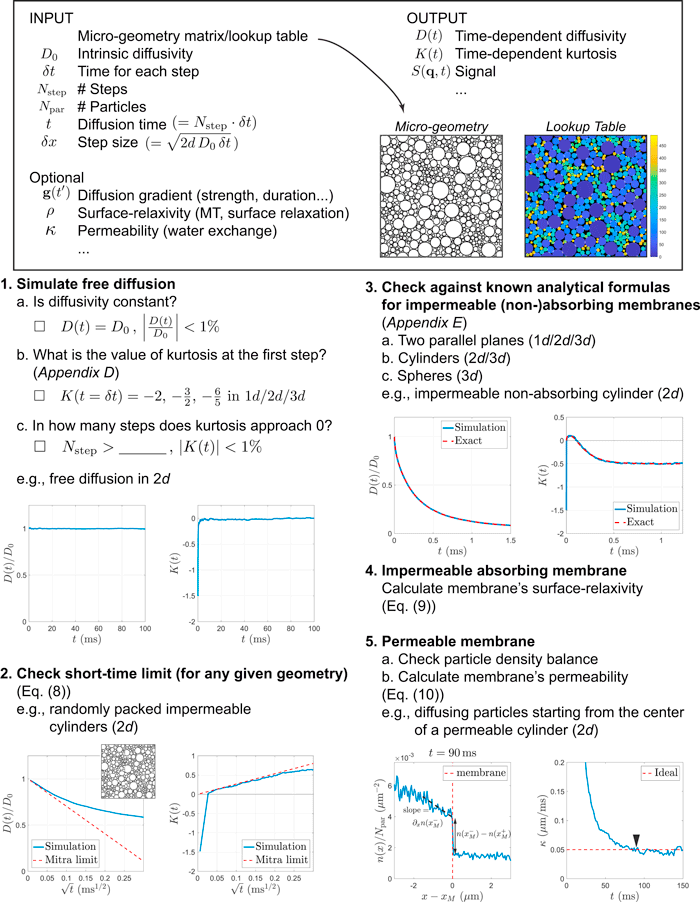Categories

# Monte Carlo Simulation Recipes in MATLAB

Scripts for two-dimensional modeling of diffusion.

We are making available code for Monte Carlo simulations of two-dimensional water diffusion in environments ranging from simple geometric shapes to realistic micro-geometries of biological tissue.Overview of simulations from the related publication, “Physical and numerical phantoms for the validation of brain microstructural MRI: A cookbook.”

The MATLAB code contains recipes for the following exercises:

• Simulation of diffusion in 2D (illustrated in section 1. of the figure above)
• Checking the short-time limit of diffusion in a geometry composed of randomly packed impermeable cylinders in 2D (illustrated in section 2. of the figure above)
• Checking against known analytical formulas for diffusion within an impermeable non-absorbing cylinder in 2D (illustrated in section 3. of the figure above)
• Calculating a membrane’s permeability by starting diffusing particles from the center of the permeable cylinders in 2D (illustrated in section 4. of the figure above)
• Analytical solution of time-dependent diffusivity and kurtosis between parallel planes, inside cylinders, and inside spheres.
• Generation of randomly packed cylinders while tuning axonal water fraction, inner diameter distribution, and g-ratio. (Packing geometry is generated as in Donev et al. 2005; Axonal diameter histogram comes from Aboitiz et al. 1992.)